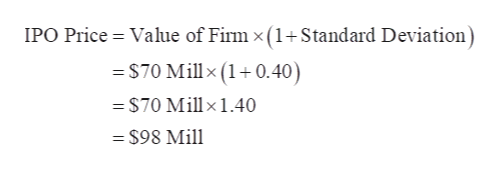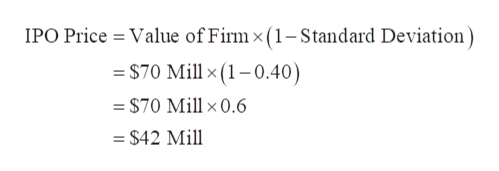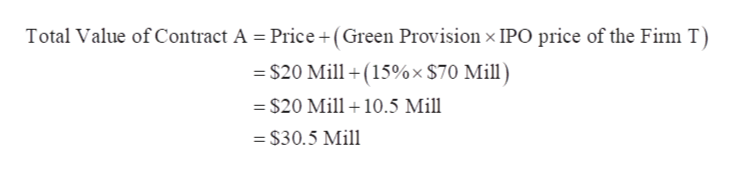# You work for Goldman Sachs who is the lead underwriter of Firm T's IPO. You need to set up the contract and Firm T has agreed to any of the two following contracts:Contract A: \$20 mill and a green provision of 15% (which matures in 1 day)Contract B: \$21mill Contract C: \$19mill +1% of the IPO priceYou have estimated the price of Firm T to be 70mill and this is the IPO price. The daily volatility on the IPO day is around 40%. The risk-free rate is 0%. Use Black-Scholes to choose one contract.Answer choices:A) Contract BB) Contract CC) You are indifferentD) Contract A

Question

You work for Goldman Sachs who is the lead underwriter of Firm T's IPO. You need to set up the contract and Firm T has agreed to any of the two following contracts:

Contract A: \$20 mill and a green provision of 15% (which matures in 1 day)

Contract B: \$21mill

Contract C: \$19mill +1% of the IPO price

You have estimated the price of Firm T to be 70mill and this is the IPO price. The daily volatility on the IPO day is around 40%. The risk-free rate is 0%. Use Black-Scholes to choose one contract.

A) Contract B
B) Contract C
C) You are indifferent
D) Contract A
check_circleExpert Solution
Step 1

Value of the Firm T= \$70 Mill

Volatility or Standard Deviation = 40% or 0.40

Value of the Firm T= \$70 Mill

Volatility or Standard Deviation = 40% or 0.40

Calculation of the IPO price of Firm T in UP situation is as follows:

Calculation of the IPO price of Firm T in UP situation is as follows:help_outlineImage TranscriptioncloseIPO Price Value of Firm x (1+Standard Deviation) -S70 Millx (1+0.40) S70 Millx1.40 = \$98 Mill fullscreen
Step 2

Calculation of the IPO price of Firm T in DOWN situation is as follows:help_outlineImage TranscriptioncloseIPO Price Value of Firm x (1- Standard Deviation = \$70 Mill x (1-0.40) = \$70 Mill x 0.6 = \$42 Mill fullscreen
Step 3

Contract A:

Price = \$20 Mill

Green provision = 15%

IPO price of the Firm T = \$70 Mill

C...help_outlineImage TranscriptioncloseTotal Value of Contract A = Price+(Green Provision x IPO price of the Firm T \$20 Mill(15%x \$70 Mill) = \$20 Mill 10.5 Mill - \$30.5 Mill fullscreen

### Want to see the full answer?

See Solution

#### Want to see this answer and more?

Solutions are written by subject experts who are available 24/7. Questions are typically answered within 1 hour*

See Solution
*Response times may vary by subject and question
Tagged in